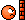# Free or cheap app for log plots

• I
Homework Helper
Gold Member
Trying to complete some homework help I got a bit stuck on an elementary or common problem that was always a bit difficult.

I have a function f(x) and just want to plot log f(x) against log x. This is common in chemistry and biochemistry. It should have been easy.I have picked up several graphing apps but they would not do it.

In the past when I occasionally needed to do this I managed with making variable 10^x or 10^-x and ugly acrobatics. It would be better to do it by a Parametric plot, and have x = log u, y = log f(u).

Whichever way you do it the trouble is the great variation in the step size. At least I think that is why it is not working. I think I may have got away with it earlier because there was not such variation of x as in my present problem, but now I need to cover at least five orders of magnitude. I think what is happening is my apps are graphing me from log x = 0 to -2 but then the whole range from -2.5 to -6 is smaller than a single step. If I made the step size small enough for that range, then it would take for ever to cover the larger range.

Anyway with the apps I have it is difficult or impossible to control the step size.
I have Free Graphing Calculator by William Jockusch. This does have parametric plotting and does havestep size control - which I found once and can't find againNice colours. Can only do four graphs but that is okay.
Have Quick Graph. More colours, more graphs, better memory, but does not have parametric that I can see.
Have a Wolfram Alpha app,, I did manage to plot the function using the 10^-x wheeze and it looks exactly as I predict, but has no nice graphics, and I wanted to put two simple (asymptote) lines on it, don't know how and saving is awkward. Did not manage to get Wolfram Alpha log plots or parametric to work at all.

Can you recommend anything? Simple to use, I really don't want to spend much time mastering complicated instructions - or lack of instructions! For the moment this is just for one homework question answer, so can't spend much time, though it will be useful in the future

Mentor

Gold Member

Excel has log axis graphing capability . Others probably do as well .

Mentor
You could also look for a MATLAB clone like freemat.org with a few lines of code get a log plot

Matlab:
x=[0:0.1:10]
y=log(x.*x)
plot(x,y)

the log function is a natural log (ie log(e) = 1) and the [0:0.1:10] creates an x array from 0 to 10 in steps of 0.1 and x.*x multiplies each element with itself to get ##x^2##

Mentor
•jedishrfu
Homework Helper
Gold Member
Thank you all for the advice - I shall look at those when I am more on top of my computer than at this moment.
It is for the moment not necessary after all. I was continually getting graphs for a simple problem that just weren't working out as they were supposed to, and I started explaining it to myself as a problems of step size. In fact it was not due to any such subtleties, I was just not writing the function right on the plotter. In fact after all the best way plot f(y) as function of log y is to let log y = x and plot f(10x) against x. So that simple thing was sorted #8 .

I had actually found that a parametric plot worked for me in Wolfram Alpha, but the figures are not very presentable, so I may be looking for parametric plotter later.